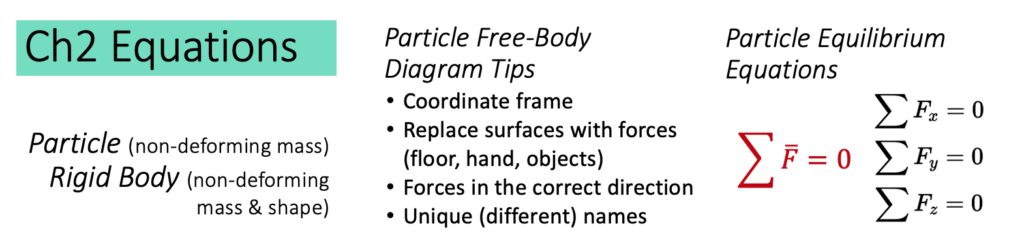# Chapter 2: Particles

In this chapter, we analyze our first static bodies (motion where acceleration = 0), treating them as particles. The sections in this chapter include:

Very simply, here are the important equations for this section (the Ch 1 equations might be helpful too)